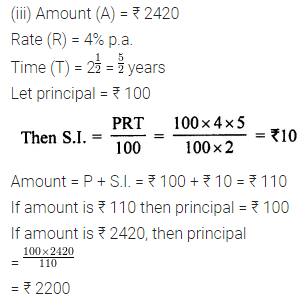# ML Aggarwal Class 7 Solutions for ICSE Maths Chapter 7 Percentage and Its Applications Ex 7.4

## ML Aggarwal Class 7 Solutions for ICSE Maths Chapter 7 Percentage and Its applications Ex 7.4

Question 1.
Find the simple interest on:
(i) ₹ 350 for 2 years at 11% per annum
(ii) ₹ 20000 for 4$$\frac { 1 }{ 2 }$$ years at 8$$\frac { 1 }{ 2 }$$ % per annum
(iii) ₹ 648 for 8 months at 16$$\frac { 2 }{ 3 }$$ % per annum. Also, find the amount in each case.
Solution: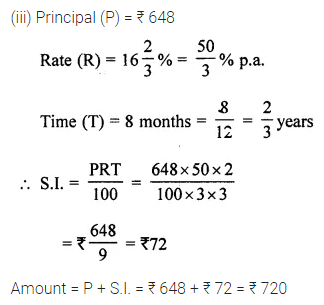Question 2.
Find the time when:
(i) simple interest on ₹ 2500 at 4% per annum is ₹ 200
(ii) simple interest on ₹ 12000 at 6$$\frac { 1 }{ 2 }$$ % per annum is ₹ 2730
Solution: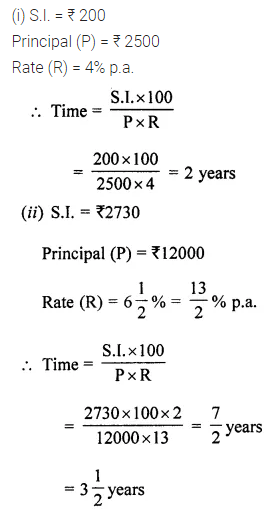Question 3.
Find the rate of interest when:
(i) simple interest on ₹ 1560 in 3 years is ₹ 585
(ii) simple interest on ₹ 1625 in 2$$\frac { 1 }{ 2 }$$ years is ₹ 325.
Solution:Question 4.
Find the principal when:
(i) simple interest at 16% per annum for 2$$\frac { 1 }{ 2 }$$ years is ₹ 3840
(ii) simple interest at 7$$\frac { 1 }{ 2 }$$ % per annum for 2 years 4 months is ₹ 2730.
Solution:Question 5.
Find the rate of interest when:
(i) ₹ 1200 amounts to ₹ 1320 in 2 years
(ii) ₹ 300 amounts to ₹ 400 in 2 years.
Solution:Question 6.
Find the time when:
(i) ₹ 1250 amounts to ₹ 1950 at 16% per annum
(ii) ₹ 6540 amounts to ₹ 8447.50 at 12$$\frac { 1 }{ 2 }$$ per annum.
Solution: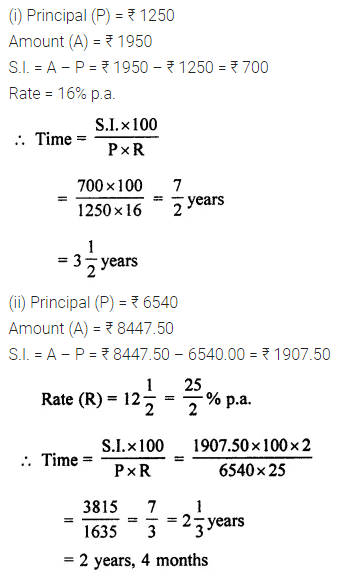Question 7.
₹ 14000 is invested at 4% per annum simple interest. How long will it take for the amount to reach ₹ 16240?
Solution: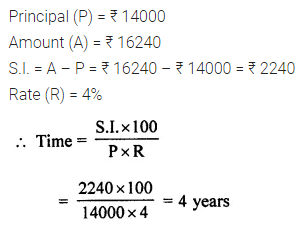Question 8.
An amount of money invested trebled in 6 years. Find the rate of interest earned.
Solution:Question 9.
Find the principal when:
(i) final amount is ₹ 4500 at 20% per annum for 5 years
(ii) final amount is ₹ 2420 at 4% per annum for 2$$\frac { 1 }{ 2 }$$ years.
Solution: